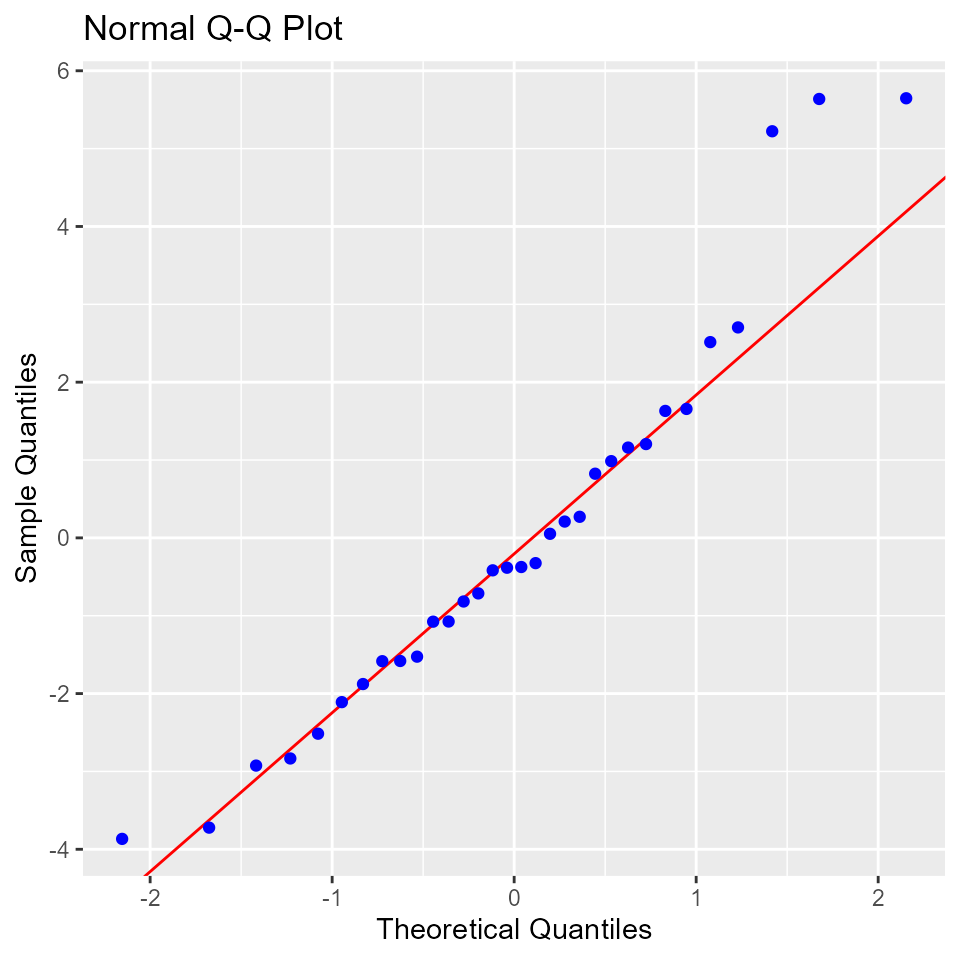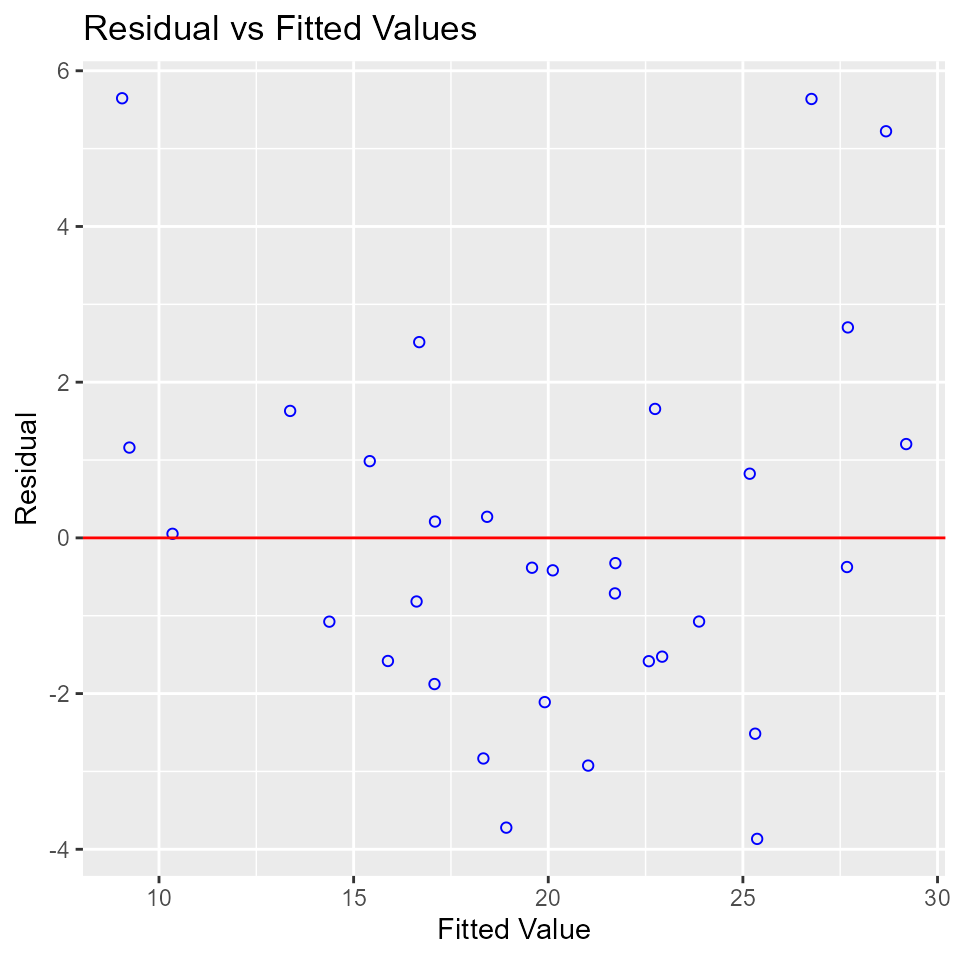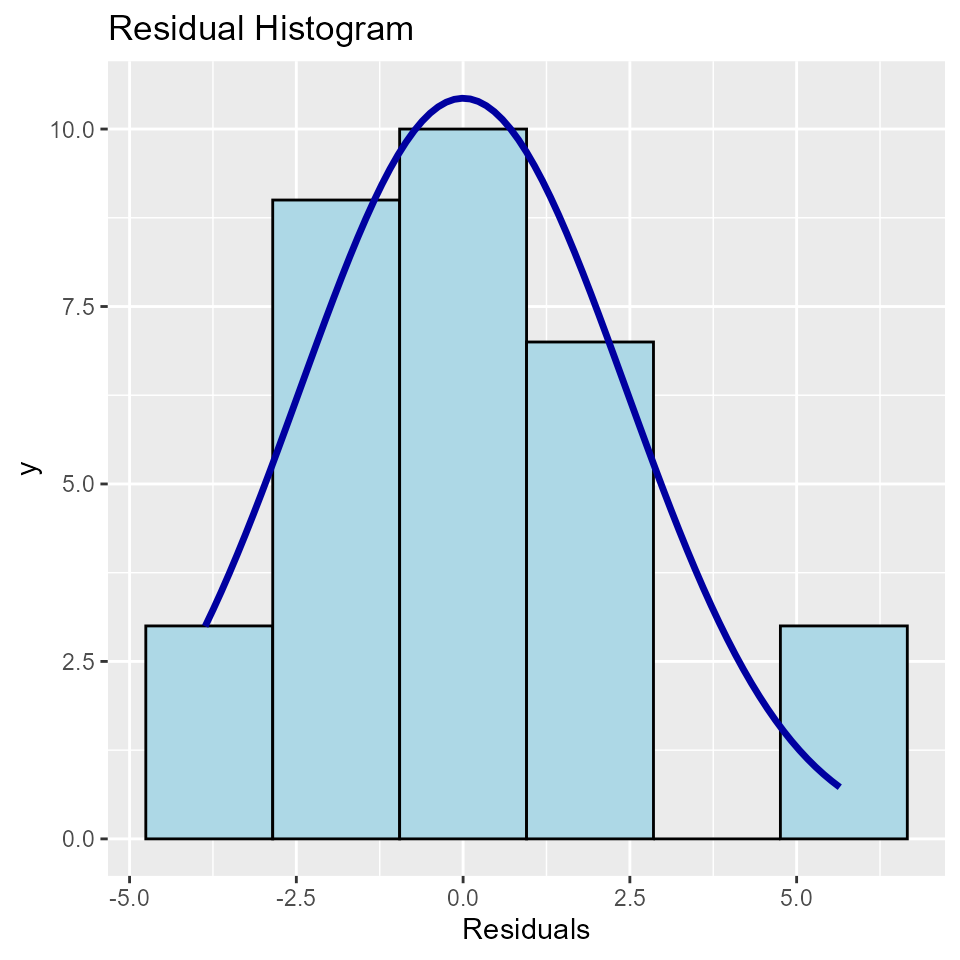## Introduction

olsrr offers tools for detecting violation of standard regression assumptions. Here we take a look at residual diagnostics. The standard regression assumptions include the following about residuals/errors:

• The error has a normal distribution (normality assumption).
• The errors have mean zero.
• The errors have same but unknown variance (homoscedasticity assumption).
• The error are independent of each other (independent errors assumption).

### Residual QQ Plot

Graph for detecting violation of normality assumption.

model <- lm(mpg ~ disp + hp + wt + qsec, data = mtcars)
ols_plot_resid_qq(model)### Residual Normality Test

Test for detecting violation of normality assumption.

model <- lm(mpg ~ disp + hp + wt + qsec, data = mtcars)
ols_test_normality(model)
## -----------------------------------------------
##        Test             Statistic       pvalue
## -----------------------------------------------
## Shapiro-Wilk              0.9366         0.0600
## Kolmogorov-Smirnov        0.1152         0.7464
## Cramer-von Mises          2.8122         0.0000
## Anderson-Darling          0.5859         0.1188
## -----------------------------------------------

Correlation between observed residuals and expected residuals under normality.

model <- lm(mpg ~ disp + hp + wt + qsec, data = mtcars)
ols_test_correlation(model)
##  0.970066

### Residual vs Fitted Values Plot

It is a scatter plot of residuals on the y axis and fitted values on the x axis to detect non-linearity, unequal error variances, and outliers.

Characteristics of a well behaved residual vs fitted plot:

• The residuals spread randomly around the 0 line indicating that the relationship is linear.
• The residuals form an approximate horizontal band around the 0 line indicating homogeneity of error variance.
• No one residual is visibly away from the random pattern of the residuals indicating that there are no outliers.
model <- lm(mpg ~ disp + hp + wt + qsec, data = mtcars)
ols_plot_resid_fit(model)### Residual Histogram

Histogram of residuals for detecting violation of normality assumption.

model <- lm(mpg ~ disp + hp + wt + qsec, data = mtcars)
ols_plot_resid_hist(model)